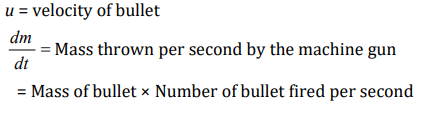## Laws of Motion Questions and Answers Part-3

1. A body of mass 40 gm is moving with a constant velocity of 2 cm/sec on a horizontal frictionless table. The force on the table is
a) 39200 dyne
b) 160 dyne
c) 80 dyne
d) Zero dyne

Explanation: Force on the table = mg = 40 * 980 = 39200 dyne

2. When 1 N force acts on 1 kg body that is able to move freely, the body receives
a) A speed of 1 m/sec
b) An acceleration of $1 m\diagup sec^{2}$
c) An acceleration of $980 cm\diagup sec^{2}$
d) An acceleration of $1 cm\diagup sec^{2}$

Explanation:3. An object with a mass 10 kg moves at a constant velocity of 10 m/sec. A constant force then acts for 4 second on the object and gives it a speed of 2 m/sec in opposite direction. The acceleration produced in it, is
a) 3 $m\diagup sec^{2}$
b) -3 $m\diagup sec^{2}$
c) 0.3 $m\diagup sec^{2}$
d) -0.3 $m\diagup sec^{2}$

Explanation:4. In the above question, the force acting on the object
a) 30 N
b) – 30 N
c) 3 N
d) – 3 N

Explanation: F = ma = 10 * (−3) = −30 N

5. In the above question, the impulse acting on the object is
a) 120 Newton $\times$ sec
b) -120 Newton $\times$ sec
c) 30 Newton $\times$ sec
d) -30 Newton $\times$ sec

Explanation: Impulse = Force * Time = −30 * 4 = −120 N-s

6. A machine gun is mounted on a 2000 kg car on a horizontal frictionless surface. At some instant the gun fires bullets of mass 10 gm with a velocity of 500 m/sec with respect to the car. The number of bullets fired per second is ten. The average thrust on the system is
a) 550 N
b) 50 N
c) 250 N
d) 250 dyne

Explanation:7. In the above question, the acceleration of the car will be
a) 0.25 $m\diagup sec^{2}$
b) 25 $m\diagup sec^{2}$
c) 5.0 $m\diagup sec^{2}$
d) 0.025 $m\diagup sec^{2}$

Explanation:8. A particle of mass 0.3 kg is subjected to a force $k=-kx$ with $k=15N\diagup m$ . What will be its initial acceleration if it is released from a point 20 cm away from the origin
a) 5 $m\diagup sec^{2}$
b) 10 $m \diagup sec^{2}$
c) 3 $m\diagup sec^{2}$
d) 15 $m\diagup sec^{2}$

Explanation:9. A block of metal weighing 2 kg is resting on a frictionless plane. It is struck by a jet releasing water at a rate of 1 kg/sec and at a speed of 5 m/sec. The initial acceleration of the block will be
a) $2.5m\diagup sec^{2}$
b) $5.0m\diagup sec^{2}$
c) $10m\diagup sec^{2}$
d) None of the above